Courses

# Subjective Questions of Electrochemistry, Past year Questions (Part - 3) - JEE Advance, Class 12 JEE Notes | EduRev

## JEE : Subjective Questions of Electrochemistry, Past year Questions (Part - 3) - JEE Advance, Class 12 JEE Notes | EduRev

The document Subjective Questions of Electrochemistry, Past year Questions (Part - 3) - JEE Advance, Class 12 JEE Notes | EduRev is a part of the JEE Course Class 12 Chemistry 35 Years JEE Mains &Advance Past yr Paper.
All you need of JEE at this link: JEE

27. How many grams of silver could be plated out on a serving tray by electrolysis of a solution containing silver in +1 oxidation state for a period of 8.0 hours at a current of 8.46 amperes? What is the area of the tray if the thickness of the silver plating is 0.00254 cm? Density of silver is 10.5 g/cm3.
(1997 - 3 Marks)

Ans:  34.02 g, 1275.6 cm2

Solution :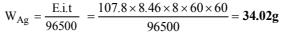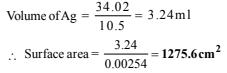28. Calculate the equilibrium constant for the reaction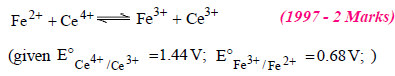Ans:  7.6 × 1012

Solution :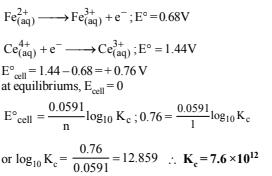29. Calculate the equilibrium constant for the reaction,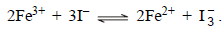The standard reduction potentials in acidic conditions are 0.77 V and 0.54 V respectively for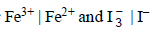couples. (1998 - 3 Marks)

Ans:  6.26 × 107

Solution :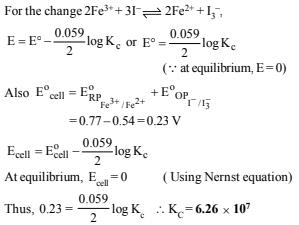30. Find the solubility product of a saturated solution of Ag2CrO4 in water at 298 K if the emf of the cell Ag | Ag+ (satd. Ag2CrO4soln.)||Ag+(0.1M)|Ag is 0.164 V at 298 K.                          (1998 - 6 Marks)

Ans:  2.44 × 10–12

Solution :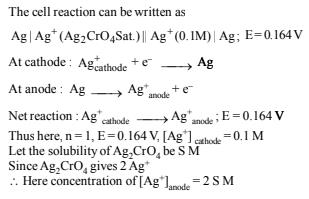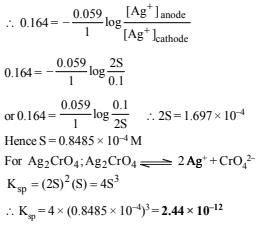31. A cell, Ag | Ag+||Cu2+|Cu, initiallly contains 1 M Ag+ and 1 M Cu2+ ions. Calculate the change in the cell potential after the passage of 9.65 A of current for 1 h. (1999 - 6 Marks)

Ans: 0.010 V

Solution :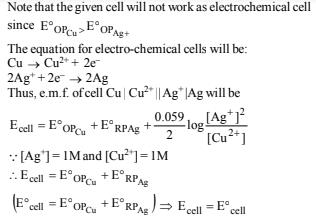After the passage of 9.65 ampere for 1 hr i.e. 9.65 x 60 x 60 Coulomb charge,during which the cell reactions are reversed, the Ag metal passes in solution state and Cu2+ ions are discharged. The reactions during the passage of current are: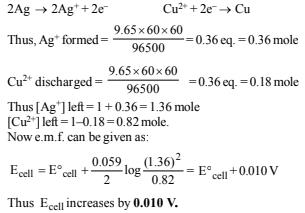32. Copper sulphate solution (250 mL) was electrolysed using a platinum anode and a copper cathode. A constant current of 2 mA was passed for 16 minutes. It was found that after electrolysis the absorbance of the solution was reduced to 50% of its original value. Calculate the concentration of copper sulphate in the solution to begin with.            (2000 - 3 Marks)

Ans:  7.95 × 10–5 mol L–1

Solution :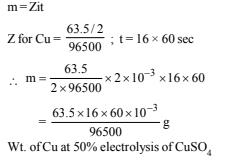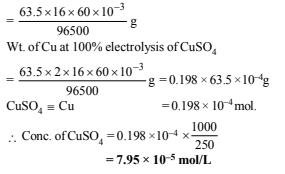33. The following electrochemical cell has been set up.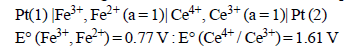If an ammeter is connected between the two platinum electrodes, predict the direction of flow of current. Will the current increase or decrease with time? (2000 - 2 Marks)

Ans:  Ce electrode to iron electrode, decrease

Solution :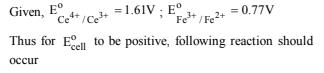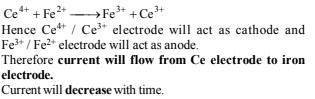34. The standard potential of the following cell is 0.23 V at 15°C and 0.21 V at 35°C.                       ( 2001 - 10 Marks)
Pt | H2 (g) | HCl(aq) | AgCl(s) | Ag(s)

(i) Write the cell reaction.
(ii) Calculate DH° and DS° for the cell reaction by assuming that these quantities remain unchanged in the range 15°C to 35°C.
(iii) Calculate the solubility of AgCl in water at 25°C.
Given : The standard reduction potential of the Ag+(aq) /Ag(s) couple is 0.80 V at 25°C.

Ans:  (ii) – 22195 J mole, –49987 J/mole; (iii) 1.24 × 10–5 mol/L

Solution :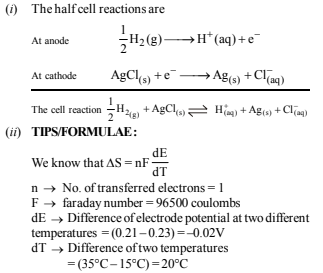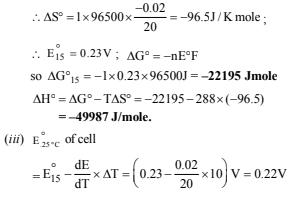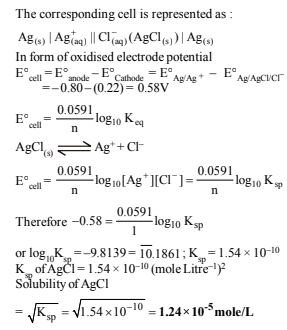35. Two students use same stock solution of ZnSO4 and a solution of CuSO4. The emf of one cell is 0.03 V higher than the other. The conc. of CuSO4 in the cell with higher emf value is 0.5 M. Find out the conc. of CuSO4 in the other cell (2.203 RT/F = 0.06). (2003 - 2 Marks)

Ans: 0.05 M

Solution :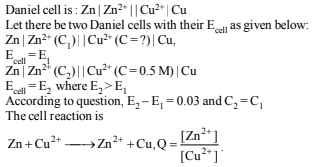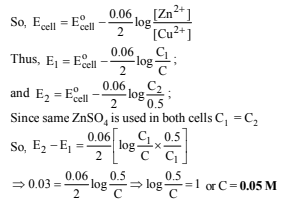36. Find the equilibrium constant for the reaction,
In2+ +   Cu2+     →    In3+ +Cu+  at  298 K
given :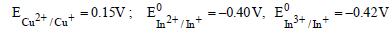(2004 - 4 Marks)

Ans: 1010

Solution :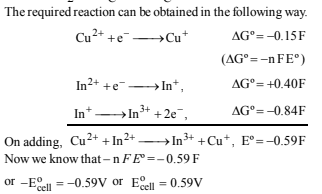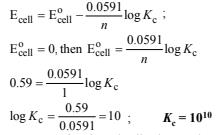37. (a) For the reaction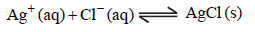Given :

 Species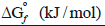Ag+ (aq) +77 Cl- (aq) -129 AgCl (s) -109

Write the cell representation of above reaction and calculate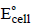at 298 K. Also find the solubility product of AgCl.

(b) If 6.539 × 10–2 g of metallic zinc is added to 100 ml saturated solution of AgCl. Find the value of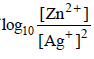How many moles of Ag will be precipitated in the above
reaction. Given that
Ag+ + e- →   Ag ; Eº = 0.80V ;
Zn2+ + 2e- → Zn ; Eº = -0.76V               (2005 - 6 Marks)
(It was given that Atomic mass of Zn = 65.39)

Ans:  (a) 0.59 V, 10–10 (b) 52.9, 10–6

Solution :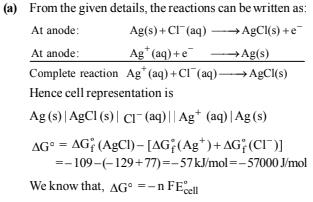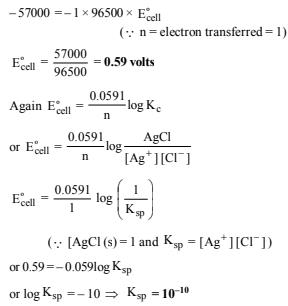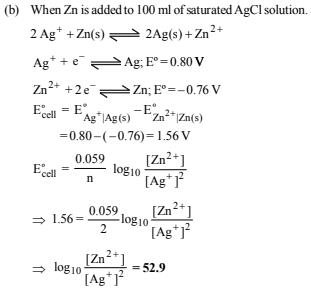NOTE: As the value of equilbrium constant is very high so the reaction moves in forward direction completely.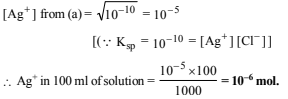38. We have taken a saturated solution of AgBr. Ksp of AgBr is 12 × 10–14. If 10–7 mole of AgNO3 are added to 1 litre of this solution find conductivity (specific conductance) of this solution in terms of 10–7 S m–1 units. Given, Molar conductance of Ag+, Br and NO3– are 6×10–3 Sm2mol–1, 8×10–3 Sm2mol–1 and 7×10–3 Sm2mol–1.

Ans: 55

Solution :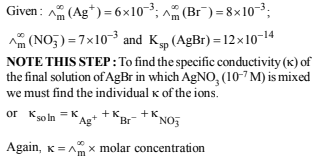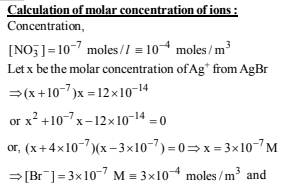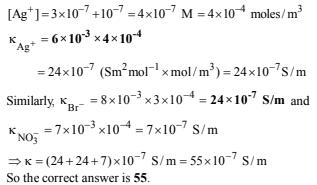Offer running on EduRev: Apply code STAYHOME200 to get INR 200 off on our premium plan EduRev Infinity!

69 docs|36 tests

,

,

,

,

,

,

,

,

,

,

,

,

,

,

,

,

,

,

,

,

,

,

,

,

,

,

,

;